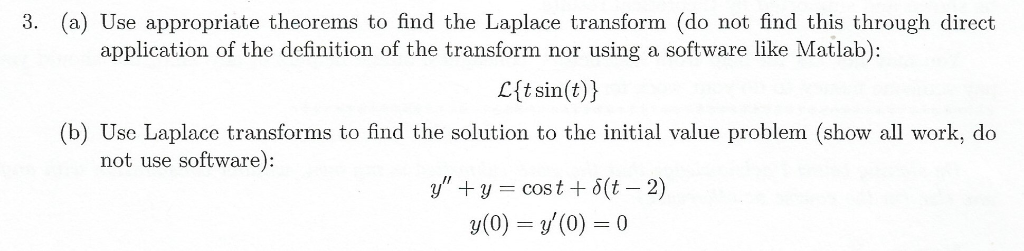# Solved: (a) Use Appropriate Theorems To Find The Laplace Transform (do Not Find This Through Direct Application Of The Definition Of The Transform Nor Using A Software Like Matlab): 3. L{tsin(t) (b) U

By |(a) Use appropriate theorems to find the Laplace transform (do not find this through direct application of the definition of the transform nor using a software like Matlab): 3. L{tsin(t) (b) Use Laplace transforms to find the solution to the initial value problem (show all work, do not use software) y ycost 5(t - 2) y(0)(0)0